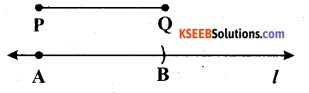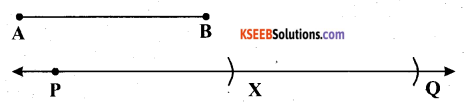# KSEEB Solutions for Class 6 Maths Chapter 14 Practical Geometry Ex 14.3

Students can Download Chapter 14 Practical Geometry Ex 14.3 Questions and Answers, Notes Pdf, KSEEB Solutions for Class 6 Maths helps you to revise the complete Karnataka State Board Syllabus and score more marks in your examinations.

## Karnataka State Syllabus Class 6 Maths Chapter 14 Practical Geometry Ex 14.3

Question 1.
Draw any line segment $$\overline{\mathbf{P Q}}$$. Without measuring $$\overline{\mathbf{P Q}}$$, construct a copy of $$\overline{\mathbf{P Q}}$$.
Solution:The following steps will be followed to draw the given line segment $$\overline{\mathbf{P Q}}$$ and to construct a copy of $$\overline{\mathbf{P Q}}$$.
(1) Let $$\overline{\mathbf{P Q}}$$ be the given line segment.
(2) Adjust the compasses up to the length of $$\overline{\mathbf{P Q}}$$.
(3) Draw any line land mark a point A on it.
(4) Put the pointer on point A, and without changing the setting of compasses, draw an arc to cut the line segment at point B.
$$\overline{\mathbf{A B}}$$ is the required line segment.Question 2.
Given some line segment $$\overline{\mathbf{A B}}$$, whose length you do not know, construct $$\overline{\mathbf{P Q}}$$ such that the length of $$\overline{\mathbf{P Q}}$$ is twice that of $$\overline{\mathbf{A B}}$$.
Solution:The following steps will be followed to construct a line segment $$\overline{\mathbf{P Q}}$$ such that the length of $$\overline{\mathbf{P Q}}$$ is twice that of $$\overline{\mathbf{A B}}$$.
(1) Let $$\overline{\mathbf{A B}}$$ be the given line segment.
(2) Adjust the compasses up to the length of $$\overline{\mathbf{A B}}$$.
(3) Draw any line 1 and mark a point P on it.
(4) Put the pointer on P and without changing the setting of compasses, draw an arc to cut the line segment at point X.
(5) Now, put the pointer on point X and again draw an arc with the same radius as before, to cut the line 1 at point Q.
$$\overline{\mathbf{P Q}}$$ is the required line segment.